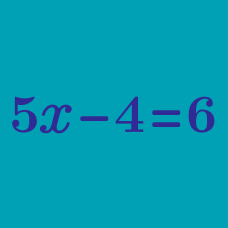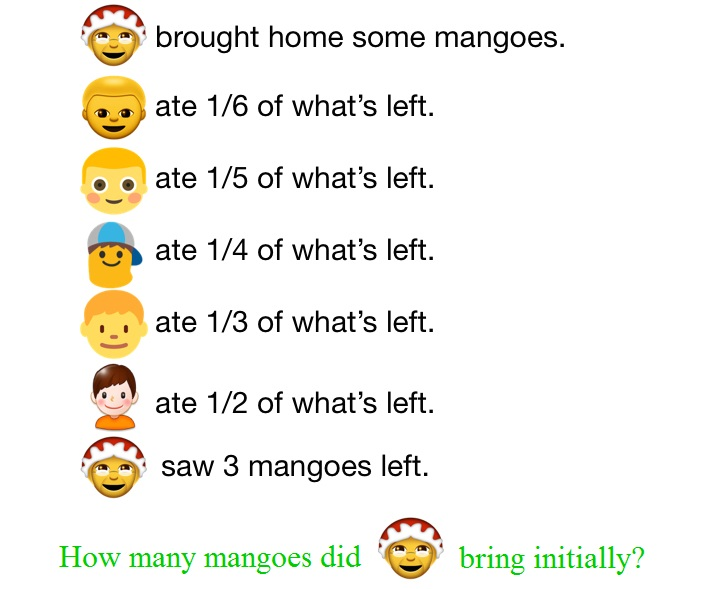Algebra

# Solving Equations: Level 2 ChallengesFive brothers stayed in the house with their mother. One day, their mother brought home some mangos.

Alex woke up first. As he was hungry, he ate $$\frac{1}{6}$$ of the mangoes, and headed out.
Brian woke up next. As he was hungry, he ate $$\frac{1}{5}$$ of what was left, and headed out.
Charles woke up next. As he was hungry, he ate $$\frac{1}{4}$$ of what was left, and headed out.
Danny woke up next. As he was hungry, he ate $$\frac{1}{3}$$ of what was left, and headed out.
Euler woke up next. As he was hungry, he ate $$\frac{1}{2}$$ of what was left, and headed out.

Their mother came home and saw 3 mangoes in the basket. How many mangoes were there initially?A teacher writes 6 consecutive integers on the blackboard.

He erases one of the integers and the sum of the remaining five integers is 2016.

What integer was erased?

There are 100 people in a room, exactly 99% are physicist. How many physicist must leave the room to bring down the percentage of physicist to exactly 98%?

True or False?

$\text{If } ac = bd \text{ and } c = d, \text{ then it is certain that } a = b.$

If we take a certain 2-digit integer and reverse its digits to form another 2-digit integer, the absolute difference between these two numbers is always divisible by which of the following numbers?

×